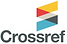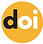top of page## ISSN 2621-5799Published: 20 December 2021

# Examining Problem-Solving and Problem-Posing Skills of Pre-Service Mathematics Teachers: A Qualitative Study

### Furkan Özdemir, Halil Coşkun Çelik

Siirt University, Turkey10.31014/aior.1993.04.04.405

Pages: 428-444

Keywords: Mathematics Education, Problem-Solving, Problem-Posing, Evaluation, Pre-Service Mathematics Teachers

Abstract

The aim of this study is to examine the problem-solving processes and problem-posing skills of pre-service mathematics teachers, which consists of four stages (understanding the problem, preparing a plan for the solution, applying the plan, evaluating) defined by Polya (1997) with the progressive scoring scale based on the alternative assessment approach. Qualitative research approach has been adopted in the study. Participants of the study consist of 71 pre-service teachers studying at the department of primary education mathematics teaching at the education faculty of a state university in the Southeastern Anatolia region of Turkey. Since the problem solving and problem posing behaviors of the participants were examined separately in the study, the gradual scoring scale developed by Baki (2008) was used. As a result of the analysis, it was determined that the participants showed the highest performance in the category of understanding the problem, and the lowest performance in the category of evaluation and problem posing. It was determined that participants who failed in the problem posing phase either wrote the same problem or could not write a problem. Another result reached in the study is that the participants had difficulties in expressing the operations in mathematical language.

References

1. Akarsu-Yakar, E., & Yılmaz, S. (2017). Mathematical language skills of 7th grade students in the process of transforming the real life situation into a mathematical expression in algebra, Inonu University Journal of the Faculty of Education, 18(1), 292-310.

2. Arıkan, E., & Ünal, H. (2013). The analysis of mathematical problem posing skill of elementary second grade students, Amasya Education Journal, 2(2), 305-325.

3. Baki, A. (2008). Kuramdan uygulamaya matematik eğitimi[Mathematics education from theory to practice]. Ankara: Harf Publishing.

4. Baki, A. (2018). Matematiği öğretme bilgisi[Knowledge of teaching mathematics]. Ankara: Pegem Academy Publishing.

5. Bonotto, C., & Santo, L. D. (2015). On the relationship between problem posing, problem solving, and creativity in the primary school. In F.M. Singer, N. F. Ellerton, & J. Cai (Ed.), Mathematical problem posing: From research to effective practice(pp. 104-121). New York, NY: Springer.

6. Brahier, D.J. (2016). Teaching secondary and middle school mathematics.New York, NY: Taylor & Francis

7. Bunar, N. (2011). The sixth graders? problem posing and solving abilities in sets, fractions and four operations (Unpublished master’s thesis). Afyon Kocatepe University, Afyon.

8. Cai, J., Hwang, S., Jiang, C., & Silber, S. (2015). Problem-posing research in mathematics education: some answered and unanswered questions. In Singer, F.M., Ellerton, N.F., & Cai, J. (Edt). Mathematical problem posing: From research to effective practice, (pp. 3-34). New York: Springer.

9. Cifarelli, V. V., & Sevim, V. (2015). Problem posing as reformulation and sense-making within problem solving. In F. M. Singer, N. F. Ellerton, & J. Cai (Ed.), Mathematical problem posing from research to effective practice (pp. 177-194). New York, NY: Springer.

10. Çepni, S. (2012). Araştırma ve proje çalışmalarına giriş [Introduction to research and project work].(6th Edition). Trabzon: Celepler Publishing.

11. Çetinkaya, A., & Soybaş, D. (2018). An investigation of problem posing skills of elementary school 8th grade students. Journal of Theoretical Educational Science, 11(1), 169-200.

12. Deringöl, Y. (2006 New approaches in the teaching of solving mathematics problems in primary education(Unpublished master’s thesis). İstanbul University, İstanbul.

13. Dinç, B. (2018). An investigation of seventh grade students' problem posing abilities for real-life situations (Unpublished master’s thesis). Eskişehir Osmangazi University, Eskişehir.

14. Ekiz, D. (2009). Araştırma yöntemleri [Research methods]. (2nd Edition). Ankara: Anı Publishing.

15. English, L. D. (2020). Teaching and learning through mathematical problem posing: Commentary. International Journal of Educational Research, 102(2020), 1-5. Doi:  https://doi.org/10.1016/j.ijer.2019.06.014

16. Ev-Çimen, E., & Yıldız, Ş. (2018). An investigation of sixth grade students’ abilities of posing problems appropriate to the column graph, Mehmet Akif Ersoy University Journal of Education Faculty, 48, 325-354.

17. Ev Çimen, E. (2018). Ability to produce strategy. InT. Kabael, (Edt.) Mathematical literacy and PISA(pp. 189-242). Ankara: Anı Publishing.

18. Gonzales, N. A. (1998). A blueprint for problem posing. School Science and Mathematics, 94(2), 78- 85.

19. Gökkurt, B. Örnek T., Hayat, F., & Soylu, Y. (2015). Assessing students’ problem-solving and problem-posing skills, Bartın University Journal of Faculty of Education, 4 (2), 751-774.

20. Jones, L. (2003). The problem with problem solving.In Ian Thompson (Edt.) Enhancing primary mathematics teaching(pp. 86-97). Maidenhead Philadelphia: Open University Press.

21. Karataş, İ., & Güven, B. (2004). Determination of 8th students’ problem solving skills: A case study. Milli Eğitim Dergisi [Journal of National Education],163.

22. Leavy, A. M., & O’Shea, J. (2011). Problem posing and pre-service primary teachers: An initial study. In T. Dooley, D. Corcoran & M. Ryan, (Eds.),Proceedings of the Fourth Conference on Research in Mathematics Education (MEI4) Theme: Mathematics Teaching Matters, 227-238, September, 22-23. Dublin, Ireland.

23. Lester Jr, F. K., & Cai, j. (2016). Can mathematical problem solving be taught? preliminary answers from 30 years of research. In P. Felmer, E. Pehkonen, & J. Kilpatrick (Ed.), Posing and solving mathematical problems advances and new perspectives(pp. 118-135). Switzerland AG: Springer.

24. Lowrie, T. (2002). Designing a framework for problem posing: Young children generating open-ended tasks. Contemporary Issues in Early Childhood, 3(3), 354-64.

25. Mayer, R. E. (1982) The psychology of mathematical problem solving. In F.K. Lester & Garofalo (Eds.), Mathematical problem solving: Issues in research(pp. 1-13). Philadelpia: Franklin Institute Press.

26. Ministry of National Education [MoNE]. (2013). Middle school mathematics (5th, 6th, 7th and 8th grades) curriculum,T.C. Ministry of National Education, Ankara.

27. Ministry of National Education [MoNE]. (2018). Mathematics curriculum (Primary and secondary school grades 1, 2, 3, 4, 5, 6, 7 and 8). T.C. Ministry of National Education, Ankara.

28. Miles, M. B., & Huberman, A. M. (1994). An expanded sourcebook: qualitative data analysis (2nd Editon). SAGE.

29. Mukhopadhyay, S., & Greer, B. (2001). Modelling with purpose: Mathematics as a critical tool. In B. Atweh, H. Forgasz, & B. Nebres (Ed.). Sociocultural research on mathematics education: An international perspective(p: 295-313). Mahwah, NJ: Lawrence Erlbaum Associates, Inc.

30. Najdowski, A. C. (2017). Flexible and focused teaching executive function skills to ındividuals with autism and attention disorders.Academic Press. Doi: 10.1016/B978-0-12-809833-2.00006-6. https://www.sciencedirect.com/science/article/pii/B9780128098332000108

31. National Council of Teacher Mathematics [NCTM.] (2000). Principles and standards for school mathematics. Reston, VA: National Council of Teacher Mathematics.

32. Norqvist, M. (2016). On mathematical reasoning - being told or finding out.Print & Media, Umeå University Umeå, Sverige. Retrieved form https://umu.diva-portal.org/smash/get/diva2:954413/FULLTEXT01.pdf

33. Polya, G. (1997). How to solve? A new dimension in mathematics.(Translated by F. Halatçı). İstanbul: Sistem Publishing.

34. Putra, H.D., Herman, T., & Sumarmo, U. (2017). Development of student worksheets to improve the ability of mathematical problem posing. International Journal on Emerging Mathematics Education, 1(1), 1-10. Doi: 10.12928/ijeme.v1i1.5507

35. Schoenfeld, A. H. (1985). Mathematical problem solving. New York: Academic Press.

36. Silver, E. A. (1994). On mathematical problem posing. For the Learning of Mathematics, 14(1), 19–28.

37. Singer, F.M., Ellerton, N.F., & Cai, J. (2015). Mathematical problem posing: From research to effective practice. New York: Springer.

38. Stickles, P. R. (2011). An analysis of secondary and middle school teachers’ mathematical problem posing. Investigations in Mathematics Learning, 3(2), 1–34. Doi: 10.1080/24727466.2011.11790301

39. Tabak, S. (2019). 6th, 7th and 8th grade students’ misconceptions about the order of operations. International Journal of Educational Methodology, 5(3), 363-373. https://doi.org/10.12973/ijem.5.3.363

40. Tall, D. (2002). Advanced mathematical thinking. New York: Kluwer Academic Publishers

41. Turhan, B. (2011). Examination of effects of mathematics teaching with problem posing approach on sixth grade students' problem solving success, problem posing abilities and views towards mathematics (Unpublished master’s thesis). Anadolu University, Eskişehir.

42. Türnüklü, E., Aydoğdu, M. Z., & Ergin, A. S. (2017). Investigation of studies an 8th grade students’ problem posing about triangles, Journal of Bayburt Education Faculty, 12(24), 467-486.

43. Uçak, A., Emir, E., Kelek, F., Kutlu, G., & Kahraman, S. (2019). Science High School 9th Grade Mathematics Textbook.Ankara: MoNE Publishing

44. Yenilmez, K., & Bağdat, O. (2014). Learning difficulties of seventh grade students about operations with integers. I. Eurasian Educational Research Congress Abstracts Booklet, İstanbul.

45. Yıldırım, A., & Şimşek, H. (2021). Sosyal bilimlerde nitel araştırma yöntemleri [Qualitative research methods in the social sciences] (12thEdition). Ankara: Seçkin Publishing.

46. Zehir, K. (2013). Investigation of pre-service elementary mathematics teachers' problem posing skills for fraction operations (Unpublished doctoral dissertation). Atatürk University, Erzurum.

bottom of page